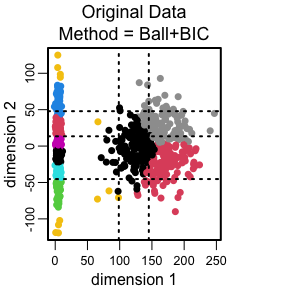# Examples of joint grid discretization

## Example 1. Nonlinear curves using kmeans+silhouette and Ball+BIC clustering with a fixed number of clusters

``````require(GridOnClusters)
x = rnorm(500)
y = sin(x)+rnorm(500, sd = 0)
z = cos(x)+rnorm(500, sd = 0)
data = cbind(x, y, z)
ks = 10

res = discretize.jointly(data, k=ks, cluster_method = "Ball+BIC",
grid_method = "Sort+split", min_level = 1)
plot(res)

res = discretize.jointly(data, k=ks, cluster_method = "kmeans+silhouette",
grid_method = "Sort+split", min_level = 1)
plot(res)``````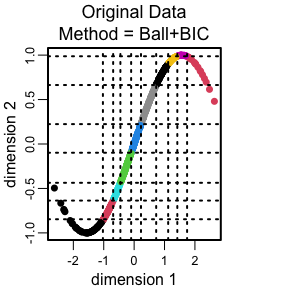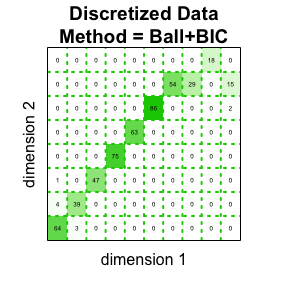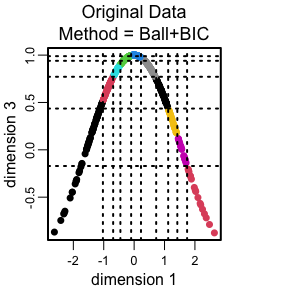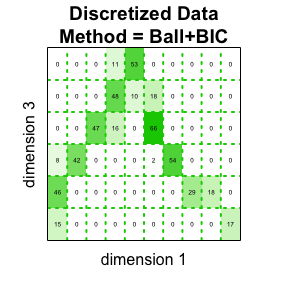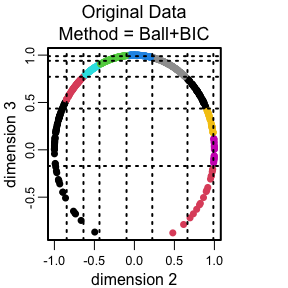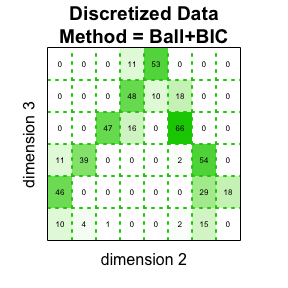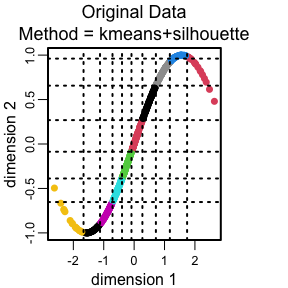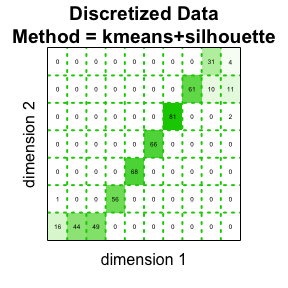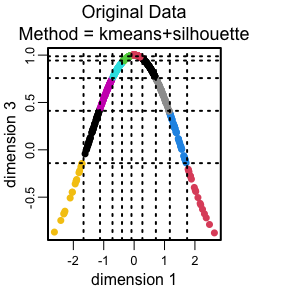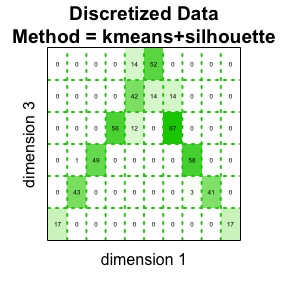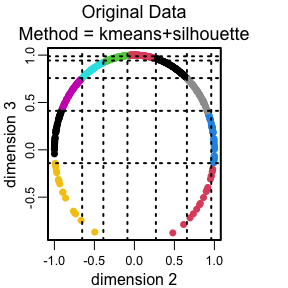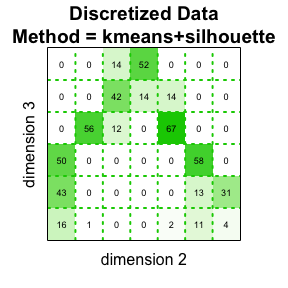Example 1. Nonlinear curves using kmeans+silhouette and Ball+BIC clustering with a fixed number of clusters.

## Example 2. Nonlinear curves and patterns using kmeans+silhouette and Ball+BIC clustering with a range for the number of clusters

``````x = rnorm(100)
y = log1p(abs(x))
z = ifelse(x >= -0.5 & x <= 0.5, 0, 1) + rnorm(100, 0, 0.1)
data = cbind(x, y, z)
ks = c(2:5)

res = discretize.jointly(data, k=ks, cluster_method = "Ball+BIC",
grid_method = "Sort+split", min_level = 1)
plot(res)

res = discretize.jointly(data, k=ks, cluster_method = "kmeans+silhouette",
grid_method = "Sort+split", min_level = 1)
plot(res)``````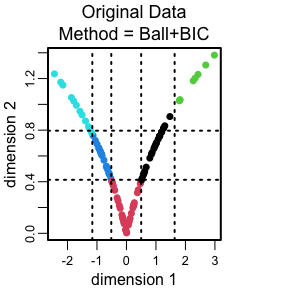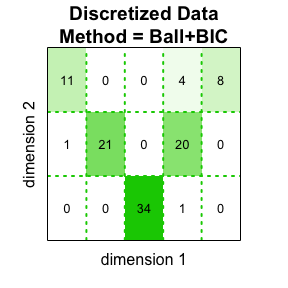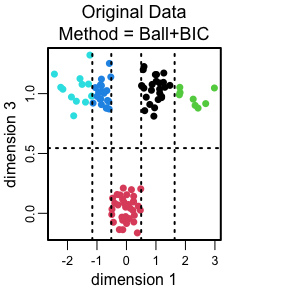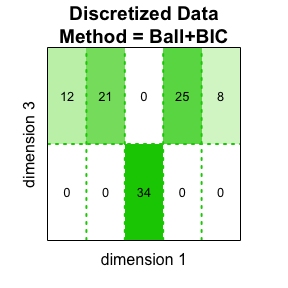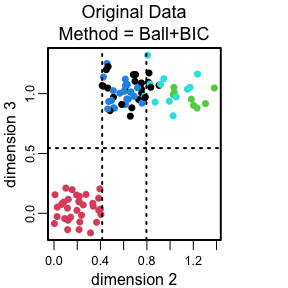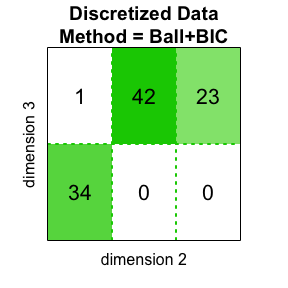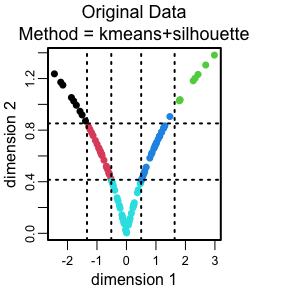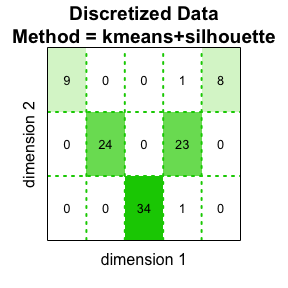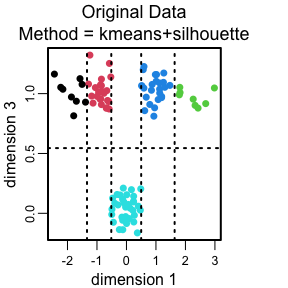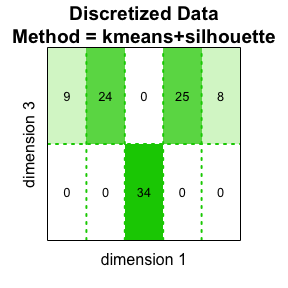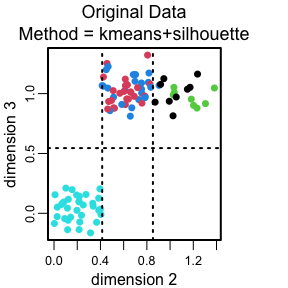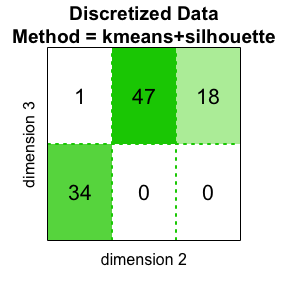Example 2. Using a range for the number of kmeans+silhouette and Ball+BIC clusters

## Example 3. Using the partition around medoids clustering method

``````# using a clustering method other than kmeans+silhouette
x = rnorm(100)
y = log1p(abs(x))
z = sin(x)
data = cbind(x, y, z)

# pre-cluster the data using partition around medoids (PAM)
cluster_label = cluster::pam(x=data, diss = FALSE, metric = "euclidean", k = 4)\$clustering

res = discretize.jointly(data, cluster_label = cluster_label,
grid_method = "Sort+split", min_level = 1)
plot(res, main="Original data\nPAM clustering",
main.table="Discretized data\nPAM & Sort+split")``````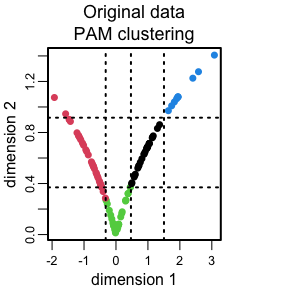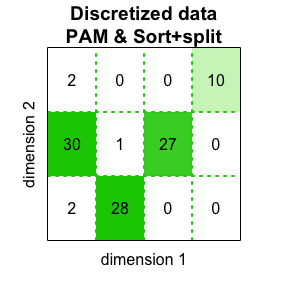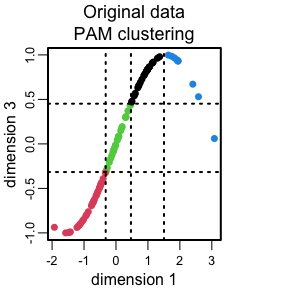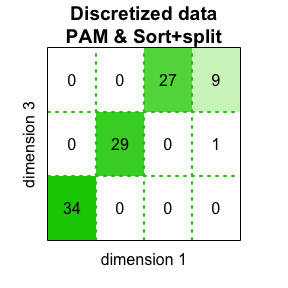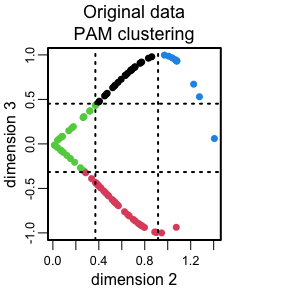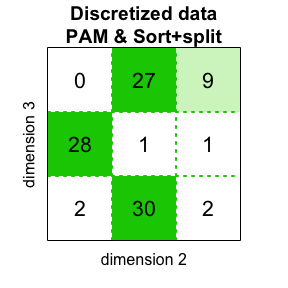Example 3. Using the partition around medoids clustering method.

## Example 4 Random patterns using kmeans+silhouette and Ball+BIC clustering with a range for the number of clusters.

``````ks = 2:20
n = 40*10
sd = 60*4

x=rnorm(2*n, sd=sd)
y=rnorm(2*n, sd=sd)
x=c(x,rnorm(2*n, sd=sd/3))
y=c(y,rnorm(2*n, sd=sd/3)+200)

data = cbind(x, y)

res = discretize.jointly(data, k=ks, cluster_method = "Ball+BIC",
grid_method = "Sort+split", min_level = 1)
plot(res)

res = discretize.jointly(data, k=ks, cluster_method = "kmeans+silhouette",
grid_method = "Sort+split", min_level = 1)
plot(res)``````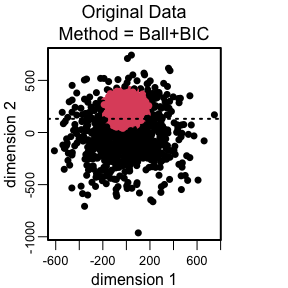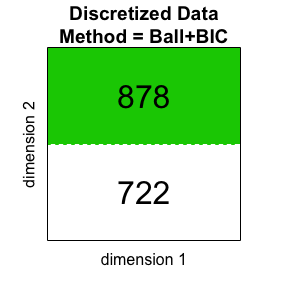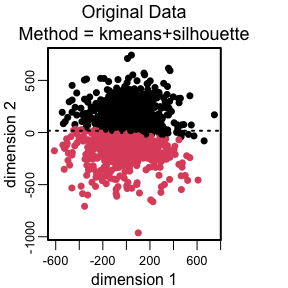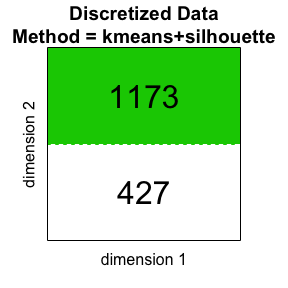Example 4. Random patterns using kmeans+silhouette and Ball+BIC clustering with a range.

## Example 5. Multi-cluster random patterns using kmeans+silhouette and Ball+BIC clustering with a range for the number of clusters.

``````n <- 50*8
ks <- 2:20

X.C1 <- matrix(
c(rnorm(n, 5, sd=2),
rnorm(n, 0, sd=40)),
ncol = 2, byrow = FALSE
)
X.C2 <- matrix(
c(rnorm(n, 70, sd=1),
rnorm(n, 0, sd=1)),
ncol = 2, byrow = FALSE
)

X.C3 <- matrix(
c(rnorm(n, 150, sd=30),
rnorm(n, 0, sd=30)),
ncol = 2, byrow = FALSE
)

data = rbind(X.C1, X.C3)

res = discretize.jointly(data, k=ks, cluster_method = "Ball+BIC",
grid_method = "Sort+split", min_level = 1)
plot(res)

res = discretize.jointly(data, k=ks, cluster_method = "kmeans+silhouette",
grid_method = "Sort+split", min_level = 1)
plot(res)``````